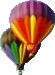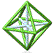Park City Mathematics Institute Number Theory and Algebra Project Abstract Drafts of Project Files (password required)

Making Nice with High School Algebra and Geometry

Very often in the classroom, a teacher will provide a question for student consumption whose answer arrives to be 3.235811 ... not a "nice" answer to most students. The objective behind the activities of our group was to provide the teacher with a mechanism to give students questions that come out "nicely", where nice generally means integers or, at least, answers with only one decimal point.

We looked at a number of question situations where having a general routine to find integer-answered questions would be convenient, including:

• Triangles with integer sides & integer areas
• Mixture problems
• Systems of equations
• Factoring
• Distance/rate/time problems
• Uniform borders around rectangles
• Law of Cosines in a integer-sided triangle
• Integer distance on the plane

Our product, then, is a series of short articles describing the problem situation and a mechanism to producing families of problems which will all result in "nice" solutions. Along with the algorithm is a description of why it actually works!

What has been most astonishing to the teachers involved is the complexity behind the questions that for most of us are remarkably routine. How many times have we solved a distance-rate-time question, or solved a system of equations? Teachers should be encouraged, and should encourage their students, to look at the general solutions to these "routine" questions and take notice of the sophisticated ideas from number theory that arise. Still waters indeed run deep.

Back to Number Theory and Algebra IndexPCMI@MathForum Home || IAS/PCMI Home© 2001 - 2018 Park City Mathematics Institute IAS/Park City Mathematics Institute is an outreach program of the School of Mathematics at the Institute for Advanced Study, Einstein Drive, Princeton, NJ 08540 Send questions or comments to: Suzanne Alejandre and Jim King